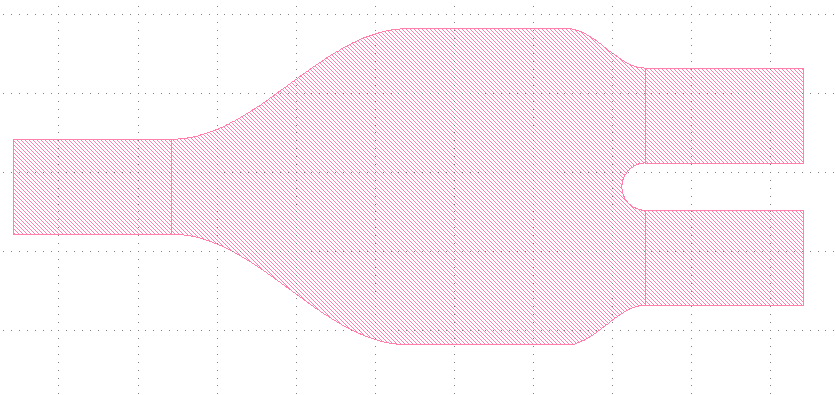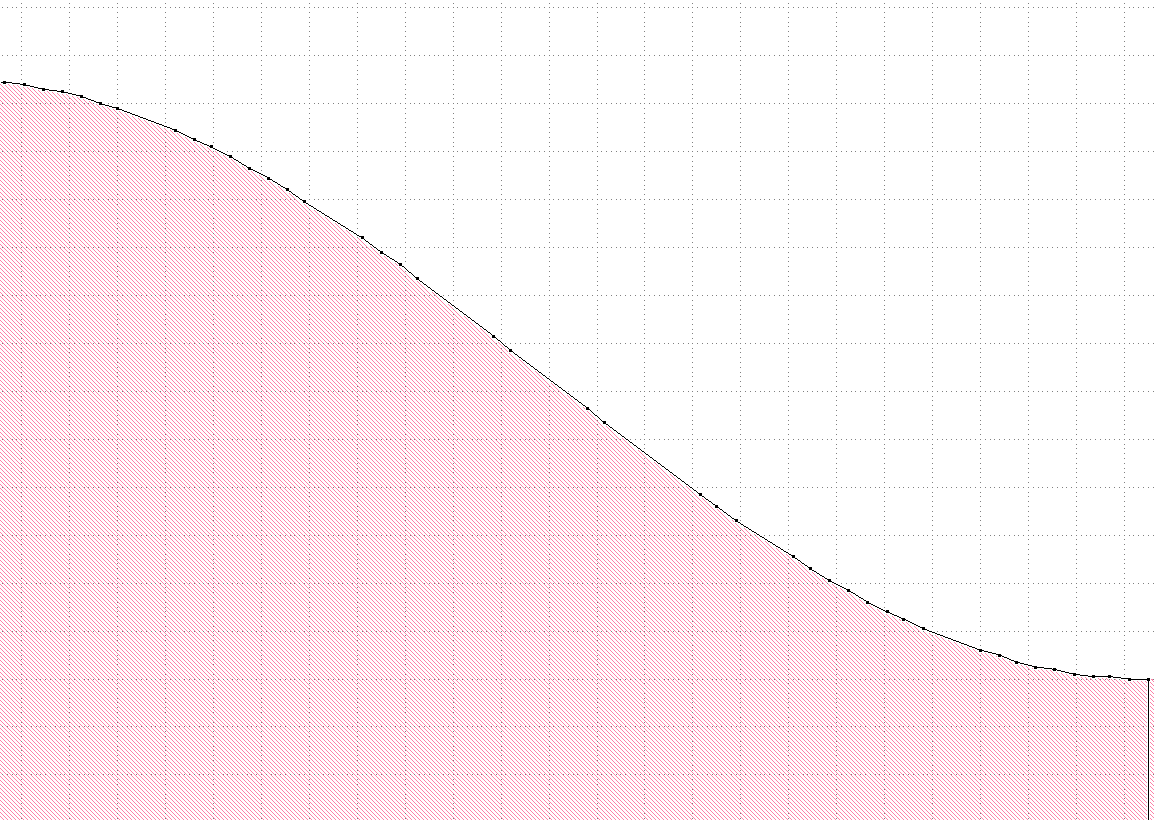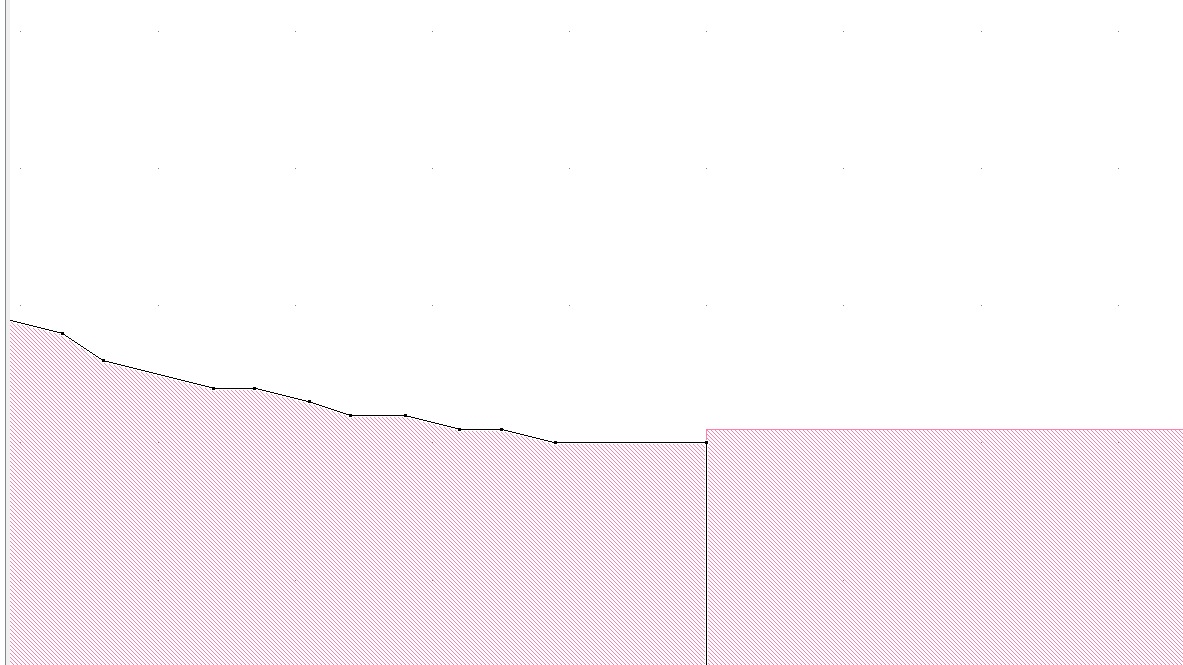# Coarse polygon border after flattening a cell

I am creating a structure having curved boundaries by first creating a PCell with python scripting.
Here is its appearance:The curved parts are simply the cosine and circle profiles and present no problem to define in the script:

`
import pya
import math

class Y_splitter(pya.PCellDeclarationHelper):

def init(self):

``````# Important: initialize the super class
super(Y_splitter, self).__init__()

# declare the parameters
self.param("l", self.TypeLayer, "Layer", default = pya.LayerInfo(1, 0))
self.param("s", self.TypeShape, "", default = pya.DPoint(0, 0))
self.param("n", self.TypeInt, "Number of points per curve", default = 64)
self.param("L1", self.TypeDouble, "L1", default = 1.5)
self.param("L2", self.TypeDouble, "L2", default = 1.0)
self.param("L3", self.TypeDouble, "L3", default = 0.5)
self.param("W", self.TypeDouble, "Width", default = 1.6)
self.param("d", self.TypeDouble, "out_wg_dist", default = 0.9)
self.param("wg_width", self.TypeDouble, "base_width", default = 0.6)
self.param("wg_length", self.TypeDouble, "wg_length", default = 1)
``````

def display_text_impl(self):
return "Y-splitter(L=" + str(self.l) + ",lambda=808 nm" + ")"

def coerce_parameters_impl(self):
pass

def can_create_from_shape_impl(self):
return False

def parameters_from_shape_impl(self):
self.r = self.shape.bbox().width() * self.layout.dbu / 2
self.l = self.layout.get_info(self.layer)

def transformation_from_shape_impl(self):
return pya.Trans( self.shape.bbox().center() )

def produce_impl(self):

``````dbu = self.layout.dbu;
ly = self.layout
shapes = self.cell.shapes

L1 = self.L1/dbu;
L2 = self.L2/dbu;
L3 = self.L3/dbu;
W = self.W/dbu;
d = self.d/dbu;
wg_width = self.wg_width/dbu;
wg_length = self.wg_length/dbu;

pts = []
dx1 = L1/self.n;
for i in range(0, self.n+1):
pts.append(pya.Point.from_dpoint(pya.DPoint(i*dx1, (W+wg_width)/4-(W-wg_width)*math.cos(math.pi*i*dx1/L1)/4  )))

dx3 = L3/self.n;
for i in range(0, self.n+1):
pts.append(pya.Point.from_dpoint(pya.DPoint(L1+L2+i*dx3, (W+wg_width+d)/4+(W-wg_width-d)*math.cos(math.pi*i*dx3/L3)/4  )))

if d>wg_width:
dphi = math.pi/2/self.n;
for i in range(0, self.n+1):

for i in range(0, self.n+1):

for i in range(self.n, -1, -1):
pts.append(pya.Point.from_dpoint(pya.DPoint(L1+L2+i*dx3, -(W+wg_width+d)/4-(W-wg_width-d)*math.cos(math.pi*i*dx3/L3)/4  )))

for i in range(self.n, -1, -1):
pts.append(pya.Point.from_dpoint(pya.DPoint(i*dx1, -(W+wg_width)/4+(W-wg_width)*math.cos(math.pi*i*dx1/L1)/4  )))

center_region = pya.Polygon(pts);
input_wg_box = pya.Box(-wg_length, -wg_width/2, 0, wg_width/2);
output_wg_poly_1 = pya.Box(L1+L2+L3, -wg_width/2+d/2, L1+L2+L3+wg_length, wg_width/2+d/2);
output_wg_poly_2 = pya.Box(L1+L2+L3, -wg_width/2-d/2, L1+L2+L3+wg_length, wg_width/2-d/2);

# create the shape
self.cell.shapes(self.l_layer).insert(center_region)
self.cell.shapes(self.l_layer).insert(input_wg_box)
self.cell.shapes(self.l_layer).insert(output_wg_poly_1)
self.cell.shapes(self.l_layer).insert(output_wg_poly_2)
``````

...

`

From geometry perspective the structure should be ok.
However, the actual appearance turns out to have roughnesses:I wonder, how can I figure out the problem.

• Hi Mikhail, typical IC layout databases have a pre-defined grid, typically 1nm. The data is stored as integer number of grid points, not real numbers. So at some point from a sine to GDSII or Oasis, the layout will be grid-snapped. I am not sure whether Oasis supports anything more sophisticated. Grids are typically "order of magnitude" numbers, e.g. 5nm or 1nm, depending on technology.

• Hi, ejprinz!

Thank you for answer. Indeed, the grid size was set to 10 nm.
However, now, after changing the grid for 1 nm in "File->Setup->Grid",
the situation is the same. I tried creating a new layout and placing the same PCell into it,
but it didn't help.
Note that if I create a circle from the basic library, its appearance is all right when flattened. The characteristic size of the curves is on the order of 100-200 nm.

Is there any way to overcome this?

• Hi Mikhail,

"grid" does not change the database unit. That's done in "File/Layout Properties".

By default the database unit is 1nm as Erwin mentioned. When you have feature with dimensions of 100-200 nm and expect a certain smoothness, you should consider going down to 0.1nm (0.0001µm).

Although the DPoint etc. objects use floating-point units these are internally converted to DBU multiples. Rounding happens by rounding to nearest. This means if you have values of lets say 100.5nm and a DBU of 1nm, the resulting coordinate may be at 100 or 101nm. A little computation error can make this difference. So as a general advice, the widths and heights you enter should be multiples of 2*DBU so the final computed coordinates round to DBU multiples.

Matthias

• Thank you, Matthias